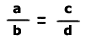# Ratio and Proportion

·       Ratio is nothing but comparison of quantities.
·       A proportion is a name we give to a statement that two ratios are equal.

It can be written in two ways:
·       Two equal fractions,or
·       Using a colon,    a:b = c:d

·       When two ratios are equal, then the cross products of the ratios are equal.
·       That is, for the proportion, a:b = c:d ,  a x d = b x c

Example:
If  A:B = 2:3 & B:C = 5:7 , then A:B:C = ?

A:B:C= 10:15:21

Points to Remember:
a : b : :  c : d
a : b  =  c : d
·       Fourth Proportion d = bc/a
·       Third Proportion c = b2/a

·       Second Proportion b = Mean Proportion = √ca
·       There is no formula for First Proportion ,Hence no question would be asked based on first proportion.Thanks for reading Ratio and Proportion

←Previous
« Prev Post
Next→
Next Post »

Video Lectures